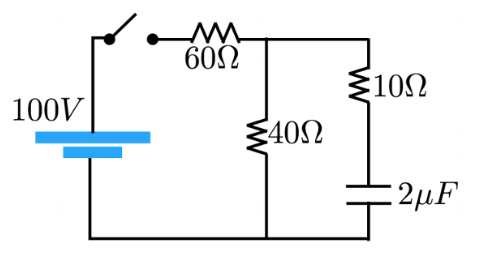# Problem: RC Circuit Analysis After the switch has been closed a very long time, what is the voltage across the capacitor?

###### FREE Expert Solution

The voltage across the 10 Ω resistor and the capacitor combination is 100 V, equal to the emf provided by the voltage source. That is:

V10Ω + Vcapacitor = 100 V

After a long time, the charging current through the branch is zero as the capacitor is fully charged.

90% (58 ratings)###### Problem Details

RC Circuit Analysis

After the switch has been closed a very long time, what is the voltage across the capacitor?Frequently Asked Questions

What scientific concept do you need to know in order to solve this problem?

Our tutors have indicated that to solve this problem you will need to apply the !! Resistor-Capacitor Circuits concept. If you need more !! Resistor-Capacitor Circuits practice, you can also practice !! Resistor-Capacitor Circuits practice problems.